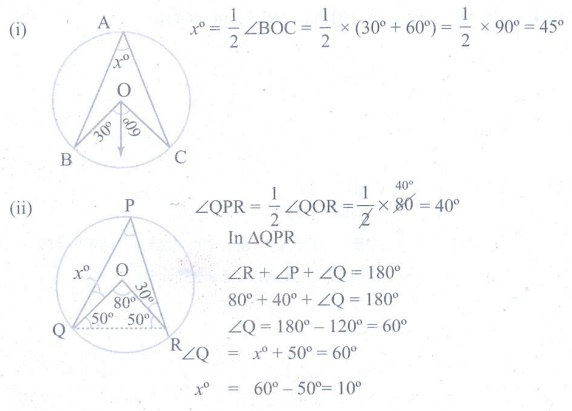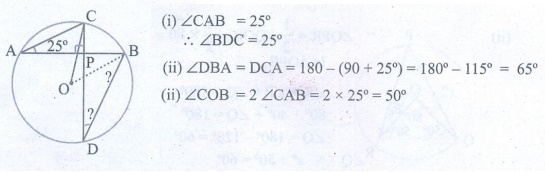Home | | Maths 9th std | Exercise 4.3: Properties of Chords of a Circle

# Exercise 4.3: Properties of Chords of a Circle

Maths : Geometry: Book Back, Exercise, Example Numerical Question with Answers, Solution: Exercise 4.3: Properties of Chords of a Circle

Properties of Chords of a Circle

Exercise 4.3

1. The diameter of the circle is 52cm and the length of one of its chord is 20cm. Find the distance of the chord from the centre.2. The chord of length 30 cm is drawn at the distance of 8cm from the centre of the circle. Find the radius of the circle3. Find the length of the chord AC where AB and CD are the two diameters perpendicular to each other of a circle with radius 4√2 cm and also find OAC and OCA.4. A chord is 12cm away from the centre of the circle of radius 15cm. Find the length of the chord.5. In a circle, AB and CD are two parallel chords with centre O and radius 10 cm such that AB = 16 cm and CD = 12 cm determine the distance between the two chords?6. Two circles of radii 5 cm and 3 cm intersect at two points and the distance between their centres is 4 cm. Find the length of the common chord.7. Find the value of x° in the following figures:8. In the given figure, CAB = 25°, find BDC , DBA and COBTags : Numerical Problems with Answers, Solution | Geometry | Maths , 9th Maths : UNIT 4 : Geometry
Study Material, Lecturing Notes, Assignment, Reference, Wiki description explanation, brief detail
9th Maths : UNIT 4 : Geometry : Exercise 4.3: Properties of Chords of a Circle | Numerical Problems with Answers, Solution | Geometry | Maths